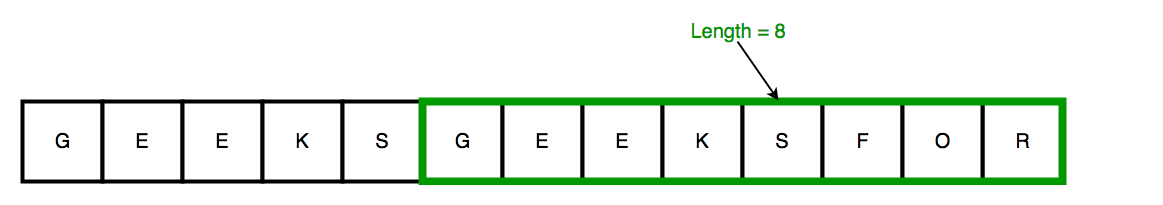Related Articles
Length of the smallest sub-string consisting of maximum distinct characters
• Difficulty Level : Hard
• Last Updated : 30 Apr, 2021

Given a string of length N, find the length of the smallest sub-string consisting of maximum distinct characters. Note : Our output can have same character.Examples:

```Input : "AABBBCBB"
Output : 5

Input : "AABBBCBBAC"
Output : 3
Explanation : Sub-string -> "BAC"

Input : "GEEKSGEEKSFOR"
Output : 8
Explanation : Sub-string -> "GEEKSFOR"```

Method 1 (Brute Force)

We can consider all sub-strings one by one and check for each sub-string both conditions together
1. sub-string’s distinct characters is equal to maximum distinct characters
2. sub-sting’s length should be minimum.

Time Complexity : O(n^3)

## C++

 `/* C++ program to find the length of the smallest``substring consisting of maximum distinct characters */``#include ``using` `namespace` `std;` `#define NO_OF_CHARS 256` `// Find maximum distinct characters in any string``int` `max_distinct_char(string str, ``int` `n){` `    ``// Initialize all character's count with 0``    ``int` `count[NO_OF_CHARS] = {0};``    ` `    ``// Increase the count in array if a character``    ``// is found``    ``for` `(``int` `i = 0; i < n;  i++)``        ``count[str[i]]++;``    ` `    ``int` `max_distinct = 0;``    ``for` `(``int` `i = 0; i < NO_OF_CHARS;  i++)``        ``if` `(count[i] != 0)     ``            ``max_distinct++;    ``    ` `    ``return` `max_distinct;``}` `int` `smallesteSubstr_maxDistictChar(string str){` `    ``int` `n = str.size();     ``// size of given string` `    ``// Find maximum distinct characters in any string``    ``int` `max_distinct = max_distinct_char(str, n);``    ``int` `minl = n;   ``// result``    ` `    ``// Brute force approach to find all substrings``    ``for` `(``int` `i=0 ;i

## Java

 `/* Java program to find the length of the smallest``substring consisting of maximum distinct characters */``class` `GFG {` `    ``final` `static` `int` `NO_OF_CHARS = ``256``;` `// Find maximum distinct characters in any string``    ``static` `int` `max_distinct_char(String str, ``int` `n) {` `        ``// Initialize all character's count with 0``        ``int` `count[] = ``new` `int``[NO_OF_CHARS];` `        ``// Increase the count in array if a character``        ``// is found``        ``for` `(``int` `i = ``0``; i < n; i++) {``            ``count[str.charAt(i)]++;``        ``}` `        ``int` `max_distinct = ``0``;``        ``for` `(``int` `i = ``0``; i < NO_OF_CHARS; i++) {``            ``if` `(count[i] != ``0``) {``                ``max_distinct++;``            ``}``        ``}` `        ``return` `max_distinct;``    ``}` `    ``static` `int` `smallesteSubstr_maxDistictChar(String str) {` `        ``int` `n = str.length();     ``// size of given string` `        ``// Find maximum distinct characters in any string``        ``int` `max_distinct = max_distinct_char(str, n);``        ``int` `minl = n;   ``// result` `        ``// Brute force approach to find all substrings``        ``for` `(``int` `i = ``0``; i < n; i++) {``            ``for` `(``int` `j = ``0``; j < n; j++) {``                ` `                ``String subs = ``null``;``                ``if``(i

## Python3

 `# Python 3 program to find the length``# of the smallest substring consisting``# of maximum distinct characters``NO_OF_CHARS ``=` `256` `# Find maximum distinct characters``# in any string``def` `max_distinct_char(``str``, n):` `    ``# Initialize all character's``    ``# count with 0``    ``count ``=` `[``0``] ``*` `NO_OF_CHARS``    ` `    ``# Increase the count in array``    ``# if a character is found``    ``for` `i ``in` `range``(n):``        ``count[``ord``(``str``[i])] ``+``=` `1``    ` `    ``max_distinct ``=` `0``    ``for` `i ``in` `range``(NO_OF_CHARS):``        ``if` `(count[i] !``=` `0``):``            ``max_distinct ``+``=` `1`   `    ` `    ``return` `max_distinct` `def` `smallesteSubstr_maxDistictChar(``str``):` `    ``n ``=` `len``(``str``)     ``# size of given string` `    ``# Find maximum distinct characters``    ``# in any string``    ``max_distinct ``=` `max_distinct_char(``str``, n)``    ``minl ``=` `n     ``# result``    ` `    ``# Brute force approach to``    ``# find all substrings``    ``for` `i ``in` `range``(n):``        ``for` `j ``in` `range``(n):``            ``subs ``=` `str``[i:j]``            ``subs_lenght ``=` `len``(subs)``            ``sub_distinct_char ``=` `max_distinct_char(subs,``                                                  ``subs_lenght)``            ` `            ``# We have to check here both conditions together``            ``# 1. substring's distinct characters is equal``            ``# to maximum distinct characters``            ``# 2. substing's length should be minimum``            ``if` `(subs_lenght < minl ``and``                ``max_distinct ``=``=` `sub_distinct_char):``                ``minl ``=` `subs_lenght` `    ``return` `minl` `# Driver Code``if` `__name__ ``=``=` `"__main__"``:``    ` `    ``# Input String``    ``str` `=` `"AABBBCBB"``    ` `    ``l ``=` `smallesteSubstr_maxDistictChar(``str``);``    ``print``( ``"The length of the smallest substring"``,``           ``"consisting of maximum distinct"``,``           ``"characters :"``, l)` `# This code is contributed by ChitraNayal`

## C#

 `/* C# program to find the length of the smallest``substring consisting of maximum distinct characters */``using` `System;``    ` `class` `GFG``{` `    ``static` `int` `NO_OF_CHARS = 256;` `    ``// Find maximum distinct characters in any string``    ``static` `int` `max_distinct_char(String str, ``int` `n)``    ``{` `        ``// Initialize all character's count with 0``        ``int` `[]count = ``new` `int``[NO_OF_CHARS];` `        ``// Increase the count in array if a character``        ``// is found``        ``for` `(``int` `i = 0; i < n; i++)``        ``{``            ``count[str[i]]++;``        ``}` `        ``int` `max_distinct = 0;``        ``for` `(``int` `i = 0; i < NO_OF_CHARS; i++)``        ``{``            ``if` `(count[i] != 0)``            ``{``                ``max_distinct++;``            ``}``        ``}` `        ``return` `max_distinct;``    ``}` `    ``static` `int` `smallesteSubstr_maxDistictChar(String str)``    ``{` `        ``int` `n = str.Length;     ``// size of given string` `        ``// Find maximum distinct characters in any string``        ``int` `max_distinct = max_distinct_char(str, n);``        ``int` `minl = n; ``// result` `        ``// Brute force approach to find all substrings``        ``for` `(``int` `i = 0; i < n; i++)``        ``{``            ``for` `(``int` `j = 0; j < n; j++)``            ``{``                ` `                ``String subs = ``null``;``                ``if``(i < j)``                    ``subs = str.Substring(i, str.Length-j);``                ``else``                    ``subs = str.Substring(j, str.Length-i);``                ``int` `subs_lenght = subs.Length;``                ``int` `sub_distinct_char = max_distinct_char(subs, subs_lenght);` `                ``// We have to check here both conditions together``                ``// 1. substring's distinct characters is equal``                ``// to maximum distinct characters``                ``// 2. substing's length should be minimum``                ``if` `(subs_lenght < minl && max_distinct == sub_distinct_char)``                ``{``                    ``minl = subs_lenght;``                ``}``            ``}``        ``}``        ``return` `minl;``    ``}` `    ``/* Driver program to test above function */``    ``static` `public` `void` `Main(String[] args)``    ``{``        ``// Input String``        ``String str = ``"AABBBCBB"``;` `        ``int` `len = smallesteSubstr_maxDistictChar(str);``        ``Console.WriteLine(``" The length of the smallest substring"``                ``+ ``" consisting of maximum distinct "``                ``+ ``"characters : "``+len);``    ``}``}` `// This code contributed by Rajput-Ji`

## PHP

 ``

## Javascript

 ``

Output:

``` The length of the smallest substring consisting
of maximum distinct characters : 5```

Method 2 (Efficient)

1. Count all distinct characters in given string.
2. Maintain a window of characters. Whenever the window contains all characters of given string, we shrink the window from left side to remove extra characters and then compare its length with smallest window fount so far.

Please refer Smallest window that contains all characters of string itself for implementation and more details.